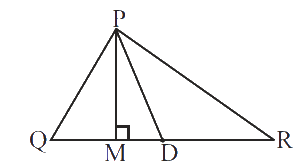# Ex.6.1 Q1 The Triangle and Its Properties - NCERT Maths Class 7

Go back to  'Ex.6.1'

## Question

In $$∆ PQR,$$ $$D$$ is the mid-point of is $$\overline {QR} \,.$$

$$\overline{PM}$$ _________________.

$$\overline{PD}$$is _________________.

Is $$QM ≠ MR?$$Video Solution
Triangle & Its Properties
Ex 6.1 | Question 1

## Text Solution

What is known:

$$PQR$$ is a triangle, $$D$$ is the mid-point of $$QR$$.

What is unknown:

What is and . Is $$QM ≠ MR?$$

Reasoning:

Let’s visually model this problem .There are three operations that done in sequence. First, check $$PM$$ is perpendicular on $$QR$$ or not,then check $$PD$$ divides $$QR$$ in equal parts or not and then find the mid-point of $$QR$$.

According to this model, the result $$PM$$ is perpendicular on $$QR$$ then $$PD$$ divides $$QR$$ in equal parts and $$D$$ is the mid-point of $$QR$$.

Steps:

Given, $$PM$$ is perpendicular on $$QR$$. Therefore, $$PM$$ is altitude.

Also, $$D$$ is the mid-point of $$QR$$

$$QD = DR$$

$$PD$$ is median

No, $$QM ≠ MR$$ , because $$D$$ is the mid-point of $$QR$$.

Learn from the best math teachers and top your exams

• Live one on one classroom and doubt clearing
• Practice worksheets in and after class for conceptual clarity
• Personalized curriculum to keep up with school